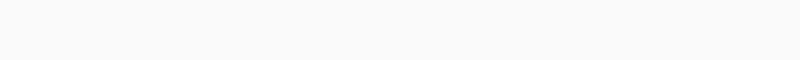# Solved – Calinski-Harabasz cluster evaluationI have used K-mean algorithm for clustering my data , and i have used Calinski-Harabasz as validity index measurement, the value of CH are :

`` k=2 , CH= 13.41,  well separated cluster   k=4 , CH= 269.68   overlapped cluster  ``

The figure is k-mean algorithm with k=2 and CH= 13.41
and the second one is k-mean algorithm with k=4 , and CH = 269.68

i have added the 3rd figure in which the cluster are not separated well and the
CH = 729 ??the K=2 and the first figure is separated well cluster , while the second one is nonseparated well cluster

any suggestion please iam confused with CH behavior.

Contents

Avoid plotting with distortion. If you plotted the data such that 1 unit on the x axis has the same size as 1 uniton the y axis, the result would likely be more comprehensible.

In your example, imagine the plot to be squeezed!compared toclearly, the bottom one does not separate the clusters well. The width of the clusters (about 40) is much larger than the separation between the cluster centers (about 2). In the top picture, thr width of the clusters is about 25,and the distance is over 10; so the top result is much better.

Don't let yourself be fooled by distorted plots!

I understand that the intuition here is different; but then you need to preprocess the data differently. As is, the distances clearly show the first result to be better.

Rate this post